# Numerical Differentiation¶

The functions described in this chapter compute numerical derivatives by finite differencing. An adaptive algorithm is used to find the best choice of finite difference and to estimate the error in the derivative.

The functions described in this chapter are declared in the header file `cml/deriv.h`.

## Functions¶

int `cml_deriv_central`(const cml_function_t *f, double x, double h, double *result, double *abserr)

This function computes the numerical derivative of the function `f` at the point `x` using an adaptive central difference algorithm with a step-size of `h`. The derivative is returned in `result` and an estimate of its absolute error is returned in `abserr`.

The initial value of `h` is used to estimate an optimal step-size, based on the scaling of the truncation error and round-off error in the derivative calculation. The derivative is computed using a 5-point rule for equally spaced abscissae at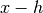,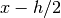,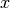,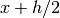,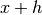, with an error estimate taken from the difference between the 5-point rule and the corresponding 3-point rule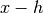,,. Note that the value of the function atdoes not contribute to the derivative calculation, so only 4-points are actually used.

int `cml_deriv_forward`(const cml_function_t *f, double x, double h, double *result, double *abserr)

This function computes the numerical derivative of the function `f` at the point `x` using an adaptive forward difference algorithm with a step-size of `h`. The function is evaluated only at points greater than `x`, and never at `x` itself. The derivative is returned in `result` and an estimate of its absolute error is returned in `abserr`. This function should be used if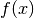has a discontinuity at `x`, or is undefined for values less than `x`.

The initial value of `h` is used to estimate an optimal step-size, based on the scaling of the truncation error and round-off error in the derivative calculation. The derivative atis computed using an “open” 4-point rule for equally spaced abscissae at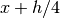,,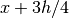,, with an error estimate taken from the difference between the 4-point rule and the corresponding 2-point rule,.

int `cml_deriv_backward`(const cml_function_t *f, double x, double h, double *result, double *abserr)

This function computes the numerical derivative of the function `f` at the point `x` using an adaptive backward difference algorithm with a step-size of `h`. The function is evaluated only at points less than `x`, and never at `x` itself. The derivative is returned in `result` and an estimate of its absolute error is returned in `abserr`. This function should be used ifhas a discontinuity at `x`, or is undefined for values greater than `x`.

This function is equivalent to calling `cml_deriv_forward()` with a negative step-size.

## Examples¶

The following code estimates the derivative of the function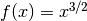at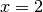and at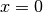. The functionis undefined for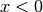so the derivative atis computed using `cml_deriv_forward()`.

```#include <stdio.h>
#include <cml.h>

double
f(double x, void *params)
{
(void)(params); /* avoid unused parameter warning */
return pow(x, 1.5);
}

int
main(void)
{
cml_function_t F;
double result, abserr;

F.function = &f;
F.params = 0;

printf("f(x) = x^(3/2)\n");

cml_deriv_central(&F, 2.0, 1e-8, &result, &abserr);
printf("x = 2.0\n");
printf("f'(x) = %.10f +/- %.10f\n", result, abserr);
printf("exact = %.10f\n\n", 1.5 * sqrt(2.0));

cml_deriv_forward (&F, 0.0, 1e-8, &result, &abserr);
printf("x = 0.0\n");
printf("f'(x) = %.10f +/- %.10f\n", result, abserr);
printf("exact = %.10f\n", 0.0);

return 0;
}
```

Here is the output of the program,

```f(x) = x^(3/2)
x = 2.0
f'(x) = 2.1213203120 +/- 0.0000005006
exact = 2.1213203436

x = 0.0
f'(x) = 0.0000000160 +/- 0.0000000339
exact = 0.0000000000
```